• Call Now

1800-102-2727•

# Valence bond theory: Postulates, potential energy diagram, Types of orbital overlap, overlapping of atomic orbitals, Extent of overlapping & Limitations

Nothing is perfect!

Have you heard that enough times in your life?

Yes, and it is correct! This belief also applies to chemistry.

If you thought the Lewis theory explained everything about compounds and molecules, you were wrong! It fails to describe many concepts like covalent bond formation and does not explain the release of energy during covalent bond formation, which is why we have the Valence Bond theory.

The structure of basic molecules was explained by Lewis theory. It did not, however, explain how chemical bonds form. In contrast, VSEPR theory explained the structure of simple molecules. Its use, however, was quite limited. It also failed to explain complex molecules' geometrical features. As a result, scientists were forced to establish the idea of valence bonds in order to address and overcome these limitations.

Table of content:

• Postulates of valence bond theory
• Potential energy diagram of hydrogen molecule
• Types of orbital overlap
• Overlapping of atomic orbitals
• Extent of overlapping
• Limitations of valence bond theory
• Practice problems

## Postulates of valence bond theory:

Pauling was the one who proposed this theory. Electrons in a molecule occupy atomic orbitals, according to the valence bond theory. The partial overlap of valence shell orbitals containing unpaired electrons forms a covalent bond. Larger the overlap, the stronger the bond will be.

1. Attractive forces exist between the nucleus of one atom and its own electron, as well as between the nucleus of one atom and the electron of another atom. Similarly, repulsive forces arise between electrons of two atoms and nucleus of two atoms.

2. The overlapping of valence orbitals containing unpaired electrons with anti-parallel spins forms covalent bonds. As a result of this overlapping, the electron density in the area between the two bonding atoms increases, boosting the stability of the resulting molecule.

3. An atom's valence shell contains unpaired electrons, allowing it to make multiple bonds with other atoms. According to the valence bond theory, the paired electrons in the valence shell do not participate in the formation of chemical bonds.

4. Covalent chemical bonds are directional in nature and are also parallel to the region corresponding to the overlapping atomic orbitals.

5. Sigma bonds and pi bonds differ in the pattern that the atomic orbitals overlap i.e.Pi bonds are formed from sidewise overlapping, whereas the overlapping along the axis that contain the nuclei of the two atoms combine to form sigma bonds. Greater the extent of the overlapping, the stronger the bond.

## Potential energy diagram for hydrogen molecule:

The intersection of half-filled atomic orbitals produces a pair of electrons pooled between the two bound atoms or molecules, according to valence bond theory. When a quota of one orbital and a quota of another orbital occupy the same area of space, we say that orbitals on two separate molecules intersect. Covalent bond effects, according to valence bond theory (VBT), when two criteria are met:

• When an orbital on one atom intersects with an orbital on another,and
• Each electron in each orbital associates to form an electron pair.

The energy of the system is determined by how closely the orbitals overlap. The graph below shows how the total energy of two hydrogen atoms vary as they get closer to each other. When the atoms are far away, there is no overlap, and we set the total of the energies to nil by convention. As the atoms get closer together, their orbitals begin to overlap. Every electron in the molecule begins to feel the attraction of another atom's nucleus.

Furthermore, the electrons and nuclei begin to repel each other respectively. While the atoms in the molecules remain widely separated, the attractions are partially stronger than the repulsions, and the system's energy tends to diminish.

As the atoms move closer together, the overlap rises, and so does the nuclei's attraction to the electrons. The energy reaches its most stable value at a specific distance between the atoms, which varies depending on how the atoms are twisted. The bond distance between the two atoms is the ideal distance between the two bonded nuclei. The bond is stable because the attractive and repulsive forces combine to provide the lowest possible energy configuration at this moment.

If the distance between the nuclei was reduced further, the repulsions between nuclei and the repulsions when electrons are restricted in closer proximity to one another would become stronger than the attractive forces. As indicated in the figure below, the energy of the structure would then rise, destabilizing the system.;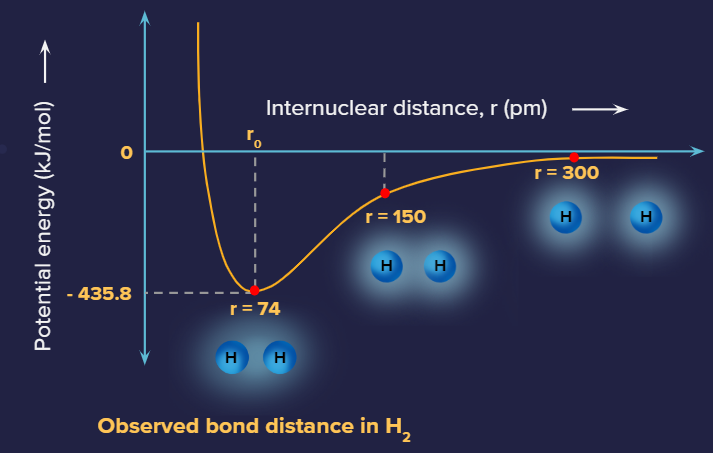Potential energy diagram of H2 molecule

As the atoms interact, the energy of the arrangement changes. The lowest (most stable) energy occurs at 74 pm, which is the bond length observed for the H2 molecule.

The bond energy is the difference between energy minimum (which occurs at the bond distance) and the energy of the two separated atoms. This is the amount of energy released when the bond forms. The same amount of energy is required to break the bond.

## Types of orbital overlap:

The covalent bond may be classified into two types depending upon the type of overlapping, i.e., sigma bond and pi bond.

Sigma () bond: This type of covalent bond is formed by head-to head overlapping of atomic orbitals along the internuclear axis. Sigma bonds are the strongest covalent bonds, owing to the direct, and hence greater extent of overlapping of the participating orbitals.The electrons involved in a sigma bond formation are often referred to as electrons. Generally, all single bonds are sigma bonds.

Types of sigma overlap:

1. s-s overlap:

One of each participating atom, s orbitals is overlapping along the internuclear axis in this type of overlapping. Before overlapping with another s orbital, it must be half-filled.

Below is an illustration of a sigma bond formed by the overlap of two s orbitals. Each hydrogen atom has a half-filled s orbital, which causes this form of overlap in H2 molecules.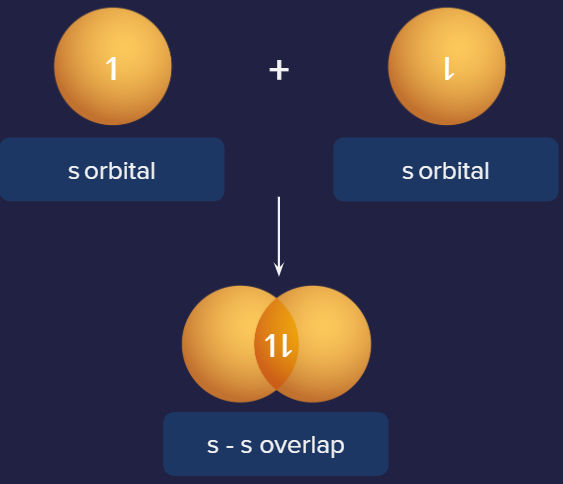Representation of s-s overlap. Example: H2

2. s-p overlap:

Along the internuclear axis, one half-filled s orbital overlaps with one half-filled p orbital, forming a covalent bond. This is depicted in the diagram below.

In Hydrochloric acid, this form of overlapping can be observed. The overlap of the p orbitals of the chlorine atom with the 1s orbitals of the hydrogen atoms forms sigma bonds in an HCl molecule.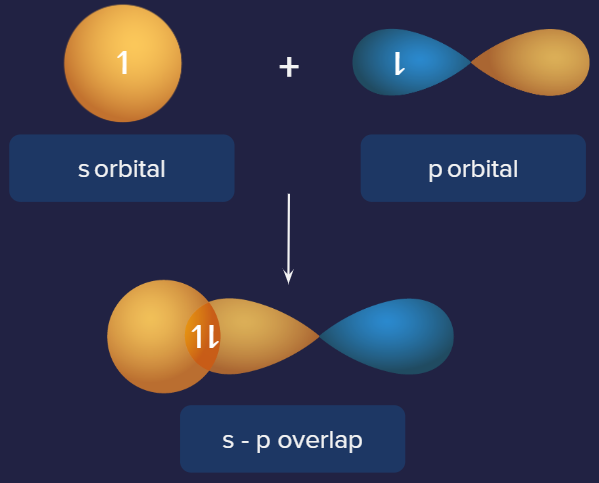Representation of s-p overlap. Example: HCl

3. p-p overlap:

One half-filled p orbital from each participating atom overlaps head to head along the internuclear axis in this condition. This type of overlapping is depicted in the diagram below.

A Cl2 molecule features a p-p overlap of the 3PZ orbitals of two chlorine atoms. It's vital to remember that overlapping two p orbitals head-to-head forms a sigma bond, but overlapping same orbitals laterally forms pi bonds.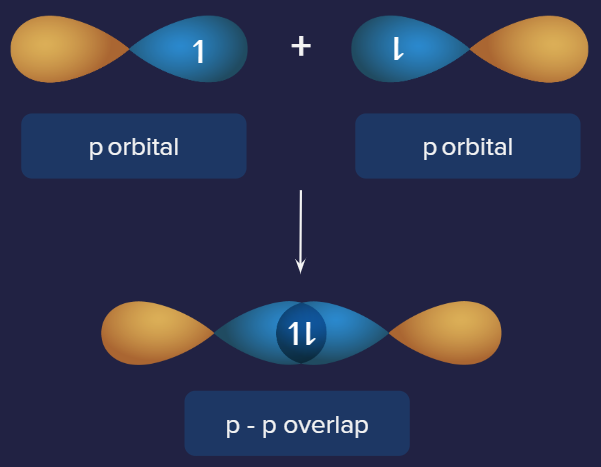Representation of p-p overlap. Example: Cl2

Pi () bond: Pi bonds are formed by the sidewise overlapping of atomic orbitals along a direction perpendicular to the internuclear axis. The axes of the atomic orbitals are parallel to each other during bond formation, while the overlapping is perpendicular to the internuclear axis.

A pi bond is formed between two atoms in addition to a sigma bond. It is usually present in molecules with multiple bonds (double or triple bonds). The electron density in pi bonds is away from the internuclear axis.

Types of pi overlap: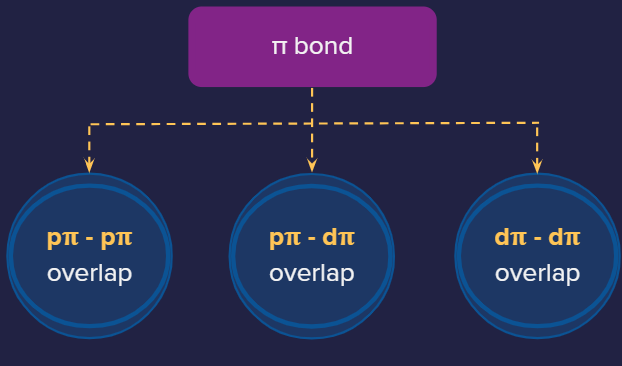1. P-P overlap: PIllustration of P-P overlap

2. P-d overlap:Illustration of P-d overlap

3. d-d overlap:Illustration of d-d overlap

## Overlapping of atomic orbitals: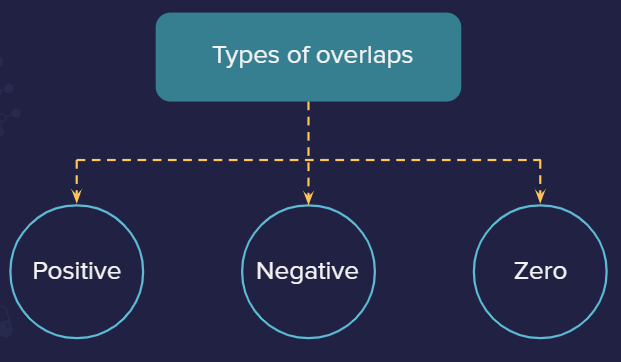There is only one phase in s-orbital (positive). There are two phases in p-orbital (positive and negative).

Positive overlap: This is when two phases overlap (positive-positive or negative-negative).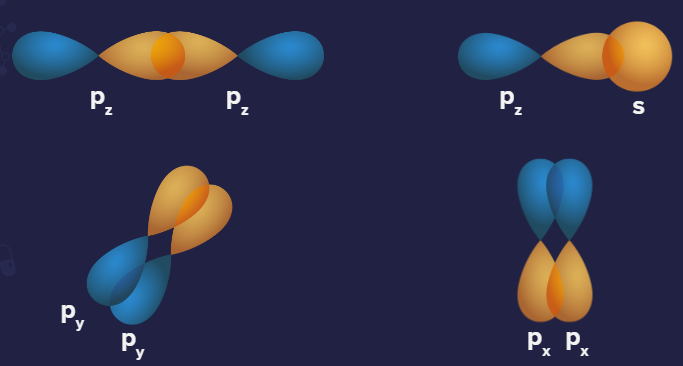Representation of positive overlapping

Negative overlap: This is when different phases overlap (positive-negative).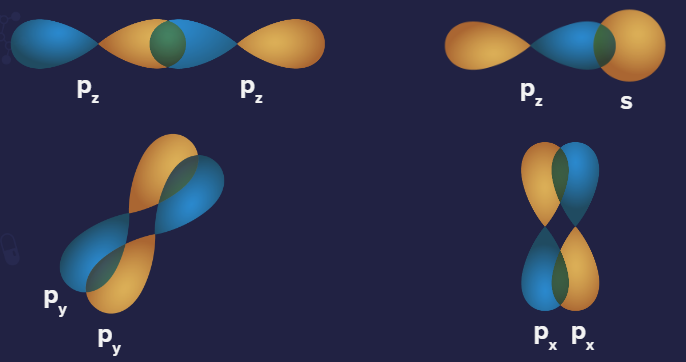Representation of negative overlapping

Zero overlap: It is when the net overlap is zero (because of equal overlap on positive and negative phases resulting in overall cancellation of each other) or no overlap occurs.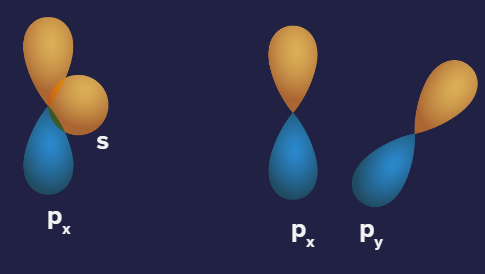Representation of Zero overlapping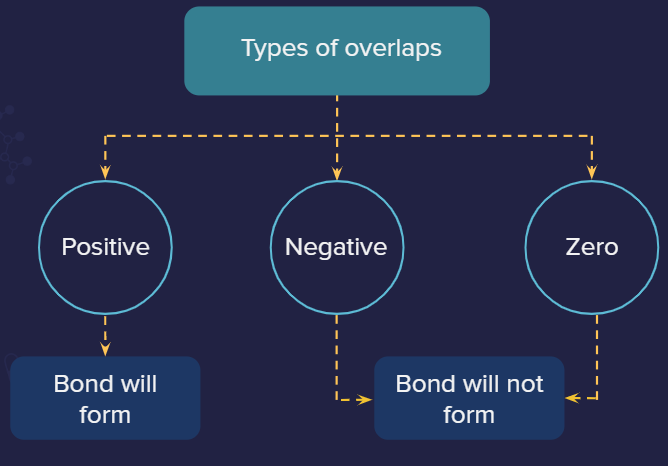Positive overlaps are allowed, negative and zero overlaps are not allowed

## Extent of overlapping:

Bond formation does not occur in all orbital overlapping. When two atoms are near enough together, their atomic orbitals overlap. Depending on the parameters of overlapping atomic orbitals, this overlap might be positive, negative, or zero. More is the extent of overlapping, stronger is the bond.

Overlapping can be divided into two categories:.

Sigma bond:

• Axial overlap
• Larger extent of overlap
• Stronger than pi bond
• For the same value of principal quantum number n, the strength of sigma overlap follows
• the order: s-s overlap < s-p overlap < p-p overlap
• For different values of principal quantum number n, the strength of sigma overlap follows the order: 3s-3s overlap < 2s-2s overlap < 1s-1s overlap

Pi bond:

• Sideways overlap
• Lower extent of overlap
• Weaker than sigma bond
• Strength of 3P-3Poverlap < 2P-2Poverlap, because 3P is more elongated as compared to 2P. So, the extent of overlap is less in 3P.

## Limitations of valence bond theory:

• It fails to explain carbon's tetravalency.
• The energies of electrons are not discussed in this theory.
• The assumptions are about the electrons being localized to specific locations.

## Practice problems:

Q.1. A positive overlap is equivalent to

(A) out-phase overlap
(B) negative overlap
(C) zero overlap
(D) in-phase overlap

Solution: Bonds are formed when there is a positive overlap. When two p-orbitals are in phase, both positive lobes overlap or both negative phase overlaps, resulting in a positive overlap and bond formation; this is called in-phase overlap.

Q.2. Which of the following does not belong to the class of homonuclear diatomic molecules?

(A) H2
(B) N2
(C) O2
(D) HCl

Solution: A homonuclear molecule is one that is formed from the atoms of the same element, while a diatomic molecule is one that is formed from the atoms of two different elements. However, because it contains different atoms, HCl is not a homonuclear diatomic molecule.

Q.3. A double bond is composed of

(A) Two sigma bonds
(B) Two pi bonds
(C) One sigma and one pi bond
(D) Two sigmas and one pi bond

Solution: A double bond is created chemically by the bonding of four electrons between two atoms. One sigma bond and one pi bond combine to form a double bond. Double bonds are involved in the production of molecules such as ethene and oxygen.

Q.4. H2O and OH have bond enthalpies of 502 KJ mol-1 and 427 KJ mol-1, respectively. What is the average bond enthalpy?

(A) 502 KJ mol-1
(B) 464.5 KJ mol-1
(C) 427 KJ mol-1
(D) 75 KJ mol-1

Solution: We know that

and

So the average bond enthalpy is given by their mean, that is . This method is used for polyatomic molecules like water.

1. Why is the sigma bond stronger than the pi bond?
Because the orbitals of a sigma bond are parallel to the internuclear axis, there is more effective orbital overlap than when pi bonds are formed, which are perpendicular to the internuclear axis. Because of the considerable difference in the extent of orbital overlap, the sigma bond is stronger than the pi bond.

2. Why are shorter bonds stronger?
Bond length is inversely proportional to bond strength; that is, the longer the bond length, the weaker the bond, and the shorter the bond length, the stronger the bond. In the case of shorter bonds, the atoms attached to one another through the bond tend to be closer as compared to the other atoms which have longer bonds and hence those bonds are weaker.

As the atoms in a molecule get closer together, the bond becomes more tightly packed, and breaking these bonds requires more energy. The bond strength grows as the number of electrons involved in the bond increases, and the space between them decreases. The sigma bond between two atoms, for example, is longer and weaker than the pi bond between the same atoms.

3. What is the main difference between VSEPR theory and valence bond theory?|
VSEPR theory and valence bond theory are both basic theories that have been created to explain the shapes and bonding of chemical species. These theories are used to explain covalently bonded molecules. The difference between VSEPR and valence bond theory is that VSEPR theory describes a molecule's shape, whereas valence bond theory explains the formation of chemical bonds between atoms in a molecule.

4. What makes ionic bonds non-directional and covalent bonds directional?
Covalent bonds are characterized as bonds established by the sharing of electrons, or by the overlapping of atomic orbitals of participant atoms. Because covalent bonds prefer specific orientations in space relative to one another, molecules have definite shape. And, in most cases, only a few patterns of overlap and spatial arrangements are possible.

For example, sigma() bonds can be formed by any one of the following types of combinations of atomic orbitals.

s-s overlapping: Overlap of two half-filled s-orbitals along the internuclear axis.

s-p overlapping: Overlap between half-filled s-orbitals of one atom and half-filled p-orbitals of another atom.

p-p overlapping: Overlap between half-filled p-orbitals of the two approaching atoms

Hence, they form bonds in a particular direction. Therefore, covalent bonds are directional in nature.

Ionic bonds, on the other hand, are different in that there is no electron sharing. When electrons are transferred from one atom to another, ionic bonds are established. Since a negative ion attracts a positive ion and vice versa. Because of this, there is a force of attraction on both sides. As a result, ionic bonds are non-directional.Talk to our expert
Resend OTP Timer =
By submitting up, I agree to receive all the Whatsapp communication on my registered number and Aakash terms and conditions and privacy policy